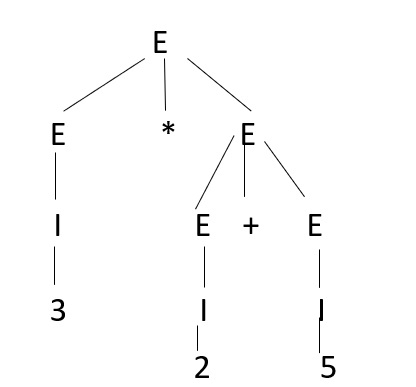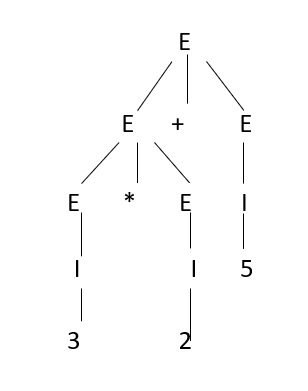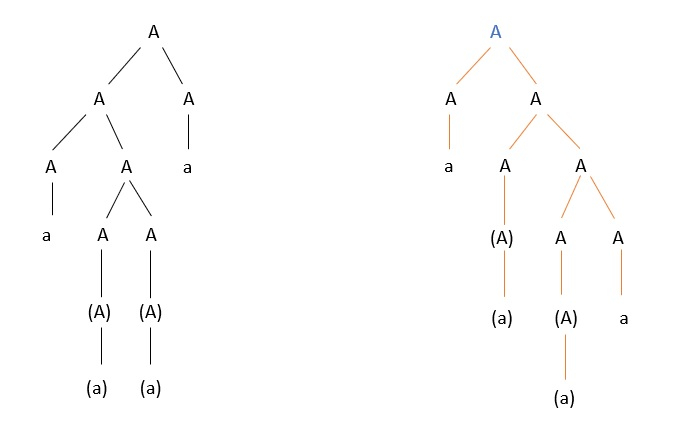# What do you mean by ambiguity in grammar in TOC?

A grammar is said to be ambiguous if there exists more than one left most derivation or more than one right most derivation or more than one parse tree for a given input string.

• If the grammar is not ambiguous then we call it unambiguous grammar.
• If the grammar has ambiguity then it is good for compiler construction.
• No method can automatically detect and remove the ambiguity, but we can remove the ambiguity by re-writing the whole grammar without ambiguity.

## Example

Let us consider a grammar with production rules, as shown below −

E = I

E = E+E

E = E*E

E = (E)

E = ε|0|1|2|3...9

Let's consider a string "3*2+5"

If the above grammar generates two parse trees by using Left most derivation (LMD) then, we can say that the given grammar is ambiguous grammar.Since there are two parse trees for a single string, then we can say the given grammar is ambiguous grammar.

Consider another example

Check whether the grammar is ambiguous or not.

A->AA

A->(A)

A->a

For the string "a(a)(a)a" the above grammar can generate two parse trees, as given below −Updated on: 12-Jun-2021

13K+ Views